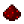# bit.blogic rshiftFunction bit.blogic_rshift Shifts a number right logically by a specified number of bits Syntax bit.blogic_rshift(number n, number bits) Returns number the value of n shifted right by bits bits, with the shifted-in bits all being zero, which is equivalent to ⌊n÷2bits⌋ for nonnegative numbers Part of ComputerCraft API bitExample Shift the number 73 (1001001) right by 2 bits, yielding 18 (10010) Code ```print(bit.blogic_rshift(73, 2)) ``` Output 18Example Shift the number 2,147,483,648 (10000000000000000000000000000000) right by 2 bits, yielding 536,870,912 (00100000000000000000000000000000) Code ```print(bit.blogic_rshift(2147483648, 2)) ``` Output 536870912